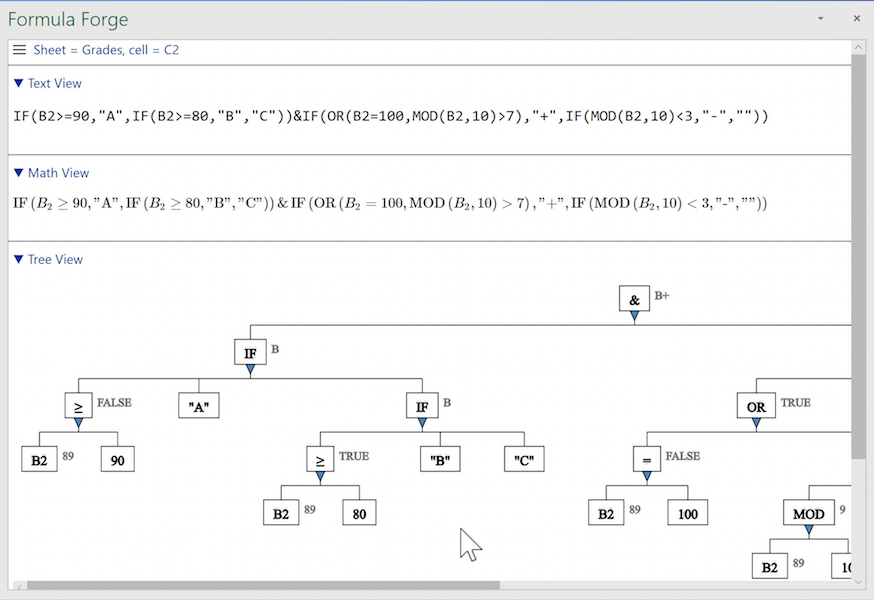# Use Cases

## Use Case: Examining a Long Formula

Long formulas can be hard to read. Consider, for example, a spreadsheet that uses a formula such as this to compute a letter grade for students scoring above average on an exam.

`IF(B2>=90,"A",IF(B2>=80,"B","C"))&IF(OR(B2=100,MOD(B2,10)>7),"+",IF(MOD(B2,10)<3,"-",""))`

It's hard to tell which parentheses match up in a formula this long, and it's hard to understand what's going on in one part of the formula when details in another part overwhelm what we want to see there. Furthermore, a formula of this size does not fit tidily into the Formula Forge pane.A long formula in a narrow pane

We can pan around in the Formula Forge pane using scrollbars (which are unfortunately somewhat elusive in Excel for Windows). Or we can try widening the Formula Forge pane by dragging its left edge further left, but the pane may still be too narrow (and Excel for the web doesn't let us widen the pane). Or we can try undocking the pane by dragging its top edge left and then resizing the undocked pane by dragging its edges outward. (But Excel for the web doesn't let us undock the pane, and Excel for the Macintosh doesn't let us widen it very much.)

Fortunately, the tree view gives us another way to see just what we want. We can suppress details associated with selected elements in the view by clicking the triangular collapse/expand icons just below those elements, thereby giving us a clearer view of the remaining elements. Formula Forge automatically synchronizes the text and math views with the tree view, replacing collapsed elements in those views with ellipses (...).Collapsing an element in the tree view

By collapsing the element in our formula that computes a letter grade, `A` through `C`, we see more clearly the `IF` element that generates a plus or minus sign to follow the letter. If we wish to focus attention on the element that computes the letter grade, we can expand it by clicking its triangular collapse/expand icon (indicated by the cursor) and collapsing the tree below the other `IF` element.